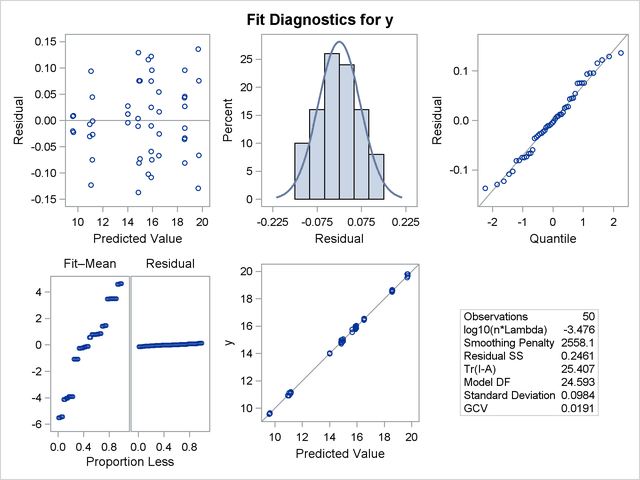# Getting Started: TPSPLINE Procedure

The following example demonstrates how you can use the TPSPLINE procedure to fit a semiparametric model.

Suppose that y is a continuous variable and x1 and x2 are two explanatory variables of interest. To fit a bivariate thin-plate spline model, you can use a MODEL statement similar to that used in many regression procedures in the SAS System:

```proc tpspline;
model y = (x1 x2);
run;
```

The TPSPLINE procedure can fit semiparametric models; the parentheses in the preceding MODEL statement separate the smoothing variables from the regression variables. The following statements illustrate this syntax:

```proc tpspline;
model y = z1 (x1 x2);
run;
```

This model assumes a linear relation with z1 and an unknown functional relation with x1 and x2.

If you want to fit several responses by using the same explanatory variables, you can save computation time by using the multiple responses feature in the MODEL statement. For example, if y1 and y2 are two response variables, the following MODEL statement can be used to fit two models. Separate analyses are then performed for each response variable.

```proc tpspline;
model y1 y2 = (x1 x2);
run;
```

The following example illustrates the use of PROC TPSPLINE. The data are from Bates et al. (1987).

```data Measure;
input x1 x2 y @@;
datalines;
-1.0 -1.0   15.54483570   -1.0 -1.0    15.76312613
-.5 -1.0   18.67397826    -.5 -1.0    18.49722167
.0 -1.0   19.66086310     .0 -1.0    19.80231311
.5 -1.0   18.59838649     .5 -1.0    18.51904737
1.0 -1.0   15.86842815    1.0 -1.0    16.03913832
-1.0  -.5   10.92383867   -1.0  -.5    11.14066546
-.5  -.5   14.81392847    -.5  -.5    14.82830425
.0  -.5   16.56449698     .0  -.5    16.44307297
.5  -.5   14.90792284     .5  -.5    15.05653924
1.0  -.5   10.91956264    1.0  -.5    10.94227538
-1.0   .0    9.61492010   -1.0   .0     9.64648093
-.5   .0   14.03133439    -.5   .0    14.03122345
.0   .0   15.77400253     .0   .0    16.00412514
.5   .0   13.99627680     .5   .0    14.02826553
1.0   .0    9.55700164    1.0   .0     9.58467047
-1.0   .5   11.20625177   -1.0   .5    11.08651907
-.5   .5   14.83723493    -.5   .5    14.99369172
.0   .5   16.55494349     .0   .5    16.51294369
.5   .5   14.98448603     .5   .5    14.71816070
1.0   .5   11.14575565    1.0   .5    11.17168689
-1.0  1.0   15.82595514   -1.0  1.0    15.96022497
-.5  1.0   18.64014953    -.5  1.0    18.56095997
.0  1.0   19.54375504     .0  1.0    19.80902641
.5  1.0   18.56884576     .5  1.0    18.61010439
1.0  1.0   15.86586951    1.0  1.0    15.90136745
;
```

The data set Measure contains three variables x1, x2, and y. Suppose that you want to fit a surface by using the variables x1 and x2 to model the response y. The variables x1 and x2 are spaced evenly on a
square, and the response y is generated by adding a random error to a function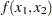. The raw data are plotted in three-dimensional scatter plot by using the G3D procedure. In order to visualize those replicates, half of the data are shifted a little bit by adding a small value (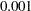) to x1 values, as in the following statements:

```data Measure1;
set Measure;
run;

proc sort data=Measure1;
by x2 x1;
run;

data Measure1;
set Measure1;
if mod(_N_, 2) = 0 then x1=x1+0.001;
run;
```
```proc g3d data=Measure1;
scatter x2*x1=y /size=.5
zmin=9 zmax=21
zticknum=4;
title "Raw Data";
run;
```

Figure 92.1 displays the raw data.

Figure 92.1 Plot of Data Set MEASURE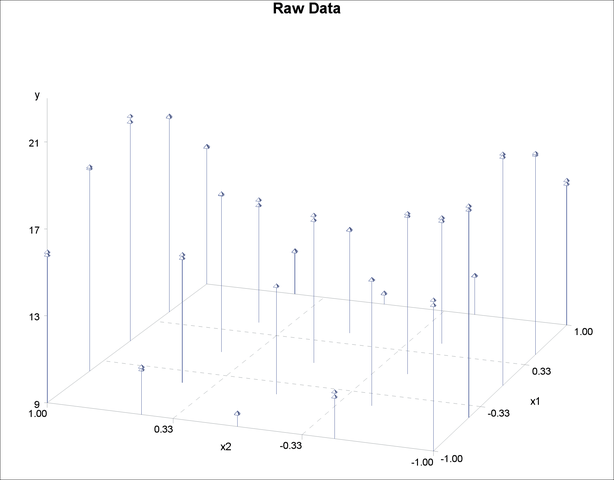The following statements invoke the TPSPLINE procedure, by using the Measure data set as input. In the MODEL statement, the x1 and x2 variables are listed as smoothing variables. The LOGNLAMBDA=  option specifies that PROC TPSPLINE examine a list of models with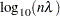ranging from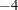to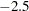. The OUTPUT statement creates the data set estimate to contain the predicted values and the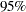upper and lower confidence limits from the best model selected by the GCV criterion.

```ods graphics on;
proc tpspline data=Measure;
model y=(x1 x2) /lognlambda=(-4 to -2.5 by 0.1);
output out=estimate pred uclm lclm;
run;

proc print data=estimate;
run;
```

When ODS Graphics is enabled, PROC TPSPLINE produces several default plots. One of the default plots is the contour plot of the fitted surface, shown in Figure 92.2. The surface exhibits nonlinear patterns along the directions of both predictors.

Figure 92.2 Fitted Surface from PROC TPSPLINE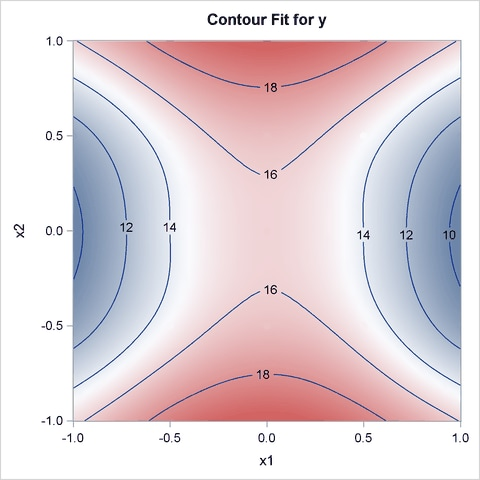Figure 92.3 shows the “Criterion Plot” that provides a graphical display of the GCV selection process. Three sets of values are shown in the plot: the specified smoothing values and their GCV values, the examined smoothing values and their GCV values during the optimization process, and the best smoothing parameter and its GCV value. The final thin-plate smoothing spline estimate is based on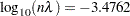, which minimizes the GCV.

Figure 92.3 The GCV Criterion by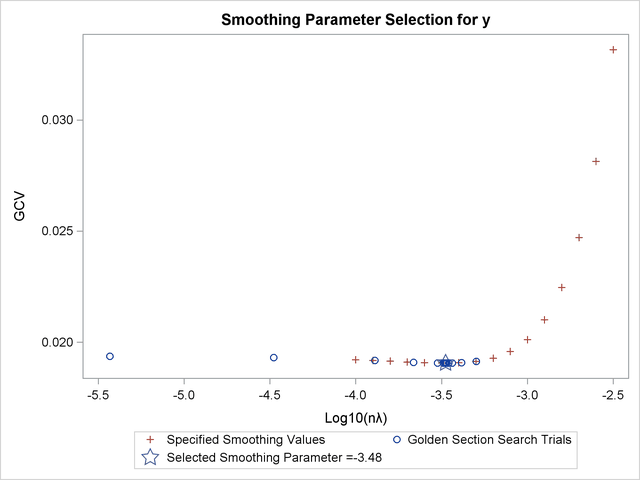Figure 92.4 shows that the data set Measure contains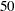observations with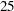unique design points. The final model contains no parametric regression terms and two smoothing variables. The order derivative in the penalty isby default, and the dimension of polynomial space is. See the section Computational Formulas for definitions.

Figure 92.4 also lists the GCV values along with the supplied values of. The value that minimizes the GCV function is
among the given list of.

The residual sum of squares from the fitted model is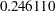, and the model degrees of freedom are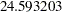. The standard deviation, defined as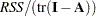, is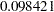. The predictions andconfidence limits are displayed in Figure 92.5.

Figure 92.4 Fitted Model Summaries from PROC TPSPLINE
 Raw Data

The TPSPLINE Procedure
Dependent Variable: y

Summary of Input Data Set
Number of Non-Missing Observations 50
Number of Missing Observations 0
Unique Smoothing Design Points 25

Summary of Final Model
Number of Regression Variables 0
Number of Smoothing Variables 2
Order of Derivative in the Penalty 2
Dimension of Polynomial Space 3

GCV Function
log10(n*Lambda) GCV
-4.000000 0.019215
-3.900000 0.019183
-3.800000 0.019148
-3.700000 0.019113
-3.600000 0.019082
-3.500000 0.019064 *
-3.400000 0.019074
-3.300000 0.019135
-3.200000 0.019286
-3.100000 0.019584
-3.000000 0.020117
-2.900000 0.021015
-2.800000 0.022462
-2.700000 0.024718
-2.600000 0.028132
-2.500000 0.033165

Note: * indicates minimum GCV value.

Summary Statistics of Final Estimation
log10(n*Lambda) -3.4762
Smoothing Penalty 2558.1432
Residual SS 0.2461
Tr(I-A) 25.4068
Model DF 24.5932
Standard Deviation 0.0984
GCV 0.0191

Figure 92.5 Data Set ESTIMATE
 Raw Data

Obs x1 x2 y P_y LCLM_y UCLM_y
1 -1.0 -1.0 15.5448 15.6474 15.5115 15.7832
2 -1.0 -1.0 15.7631 15.6474 15.5115 15.7832
3 -0.5 -1.0 18.6740 18.5783 18.4430 18.7136
4 -0.5 -1.0 18.4972 18.5783 18.4430 18.7136
5 0.0 -1.0 19.6609 19.7270 19.5917 19.8622
6 0.0 -1.0 19.8023 19.7270 19.5917 19.8622
7 0.5 -1.0 18.5984 18.5552 18.4199 18.6905
8 0.5 -1.0 18.5190 18.5552 18.4199 18.6905
9 1.0 -1.0 15.8684 15.9436 15.8077 16.0794
10 1.0 -1.0 16.0391 15.9436 15.8077 16.0794
11 -1.0 -0.5 10.9238 11.0467 10.9114 11.1820
12 -1.0 -0.5 11.1407 11.0467 10.9114 11.1820
13 -0.5 -0.5 14.8139 14.8246 14.6896 14.9597
14 -0.5 -0.5 14.8283 14.8246 14.6896 14.9597
15 0.0 -0.5 16.5645 16.5102 16.3752 16.6452
16 0.0 -0.5 16.4431 16.5102 16.3752 16.6452
17 0.5 -0.5 14.9079 14.9812 14.8461 15.1162
18 0.5 -0.5 15.0565 14.9812 14.8461 15.1162
19 1.0 -0.5 10.9196 10.9497 10.8144 11.0850
20 1.0 -0.5 10.9423 10.9497 10.8144 11.0850
21 -1.0 0.0 9.6149 9.6372 9.5019 9.7724
22 -1.0 0.0 9.6465 9.6372 9.5019 9.7724
23 -0.5 0.0 14.0313 14.0188 13.8838 14.1538
24 -0.5 0.0 14.0312 14.0188 13.8838 14.1538
25 0.0 0.0 15.7740 15.8822 15.7472 16.0171
26 0.0 0.0 16.0041 15.8822 15.7472 16.0171
27 0.5 0.0 13.9963 14.0006 13.8656 14.1356
28 0.5 0.0 14.0283 14.0006 13.8656 14.1356
29 1.0 0.0 9.5570 9.5769 9.4417 9.7122
30 1.0 0.0 9.5847 9.5769 9.4417 9.7122
31 -1.0 0.5 11.2063 11.1614 11.0261 11.2967
32 -1.0 0.5 11.0865 11.1614 11.0261 11.2967
33 -0.5 0.5 14.8372 14.9182 14.7831 15.0532
34 -0.5 0.5 14.9937 14.9182 14.7831 15.0532
35 0.0 0.5 16.5549 16.5386 16.4036 16.6736
36 0.0 0.5 16.5129 16.5386 16.4036 16.6736
37 0.5 0.5 14.9845 14.8549 14.7199 14.9900
38 0.5 0.5 14.7182 14.8549 14.7199 14.9900
39 1.0 0.5 11.1458 11.1727 11.0374 11.3080
40 1.0 0.5 11.1717 11.1727 11.0374 11.3080
41 -1.0 1.0 15.8260 15.8851 15.7493 16.0210
42 -1.0 1.0 15.9602 15.8851 15.7493 16.0210
43 -0.5 1.0 18.6401 18.5946 18.4593 18.7299
44 -0.5 1.0 18.5610 18.5946 18.4593 18.7299
45 0.0 1.0 19.5438 19.6729 19.5376 19.8081
46 0.0 1.0 19.8090 19.6729 19.5376 19.8081
47 0.5 1.0 18.5688 18.5832 18.4478 18.7185
48 0.5 1.0 18.6101 18.5832 18.4478 18.7185
49 1.0 1.0 15.8659 15.8761 15.7402 16.0120
50 1.0 1.0 15.9014 15.8761 15.7402 16.0120

You can also use the TEMPLATE and SGRENDER procedures to create a perspective plot for visualizing the fitted surface. Because the data in the data set Measure are very sparse, the fitted surface is not smooth. To produce a smoother surface, the following statements generate the data set pred in order to obtain a finer grid. The LOGNLAMBDA0= option requests that PROC TPSPLINE fit a model with a fixedvalue of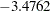. The SCORE statement evaluates the fitted surface at those new design points.

```data pred;
do x1=-1 to 1 by 0.1;
do x2=-1 to 1 by 0.1;
output;
end;
end;
run;

proc tpspline data=measure;
model y=(x1 x2)/lognlambda0=-3.4762;
score data=pred out=predy;
run;

proc template;
define statgraph surface;
dynamic _X _Y _Z _T;
begingraph /designheight=360;
entrytitle _T;
layout overlay3d/rotate=120 cube=false  xaxisopts=(label="x1")
yaxisopts=(label="x2") zaxisopts=(label="P_y");
surfaceplotparm x=_X y=_Y z=_Z;
endlayout;
endgraph;
end;
run;

proc sgrender data=predy template=surface;
dynamic _X='x1' _Y='x2' _Z='P_y'
_T='Plot of Fitted Surface on a Fine Grid';
run;

```

The surface plot based on the finer grid is displayed in Figure 92.6. The plot indicates that a parametric model with quadratic terms of x1 and x2 provides a reasonable fit to the data.

Figure 92.6 Plot of TPSPLINE Fit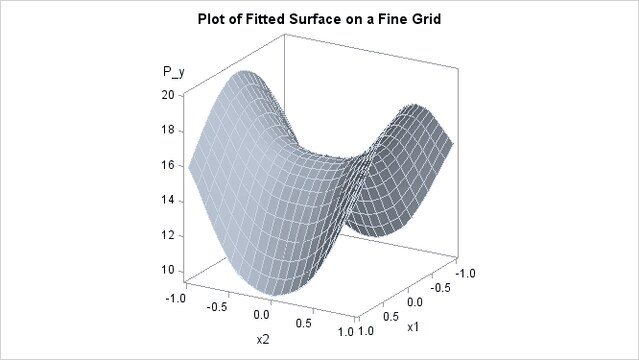Figure 92.7 shows a panel of fit diagnostics for the selected model that indicate a reasonable fit:

• The predicted values closely approximate the observed values.

• The residuals are approximately normally distributed and do not show obvious systematic patterns.

• The RFPLOT shows that much variation in the response variable is addressed by the fit and only a little remains in the residuals.

Figure 92.7 Fit Diagnostics